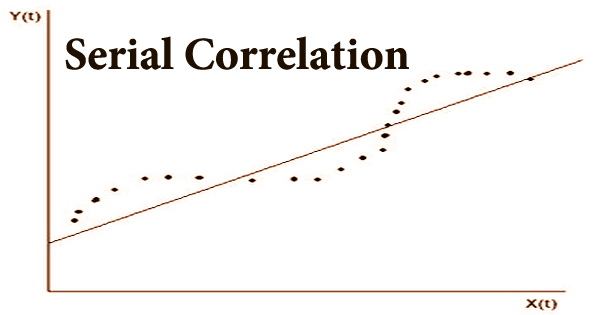### Estimation Theory – a Branch of Statistics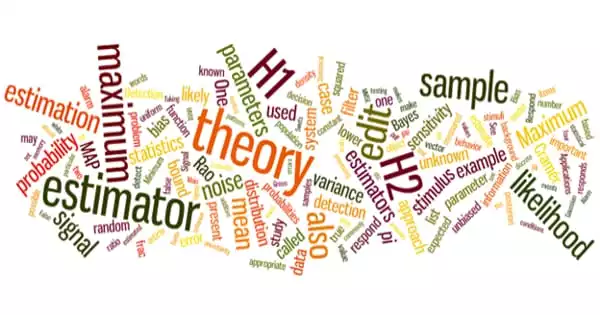Estimation theory is a branch of statistics concerned with estimating parameter values based on measured empirical data with a random component. It is a crucial mathematical concept that is used in a wide range of communication and signal processing applications. The parameters describe an underl.....

### Statistical Modeling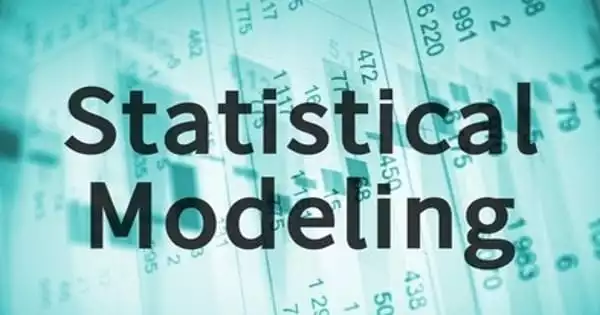Regression analysis is a set of statistical processes used in statistical modeling to estimate the relationships between a dependent variable and one or more independent variables. It is the application of statistical analysis to a dataset. Linear regression is the most common type of regression .....

### Uniform Distribution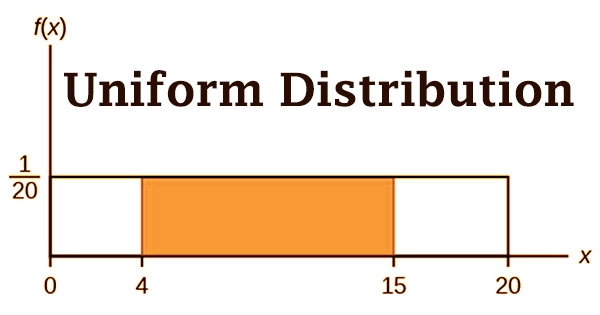Uniform distribution is a word used in statistics to describe a type of probability distribution in which every conceivable event has an equal chance of occurring. Because the chances of drawing a heart, a club, a diamond, or a spade are equal, a deck of cards has uniform distributions. The proba.....

### A Priori ProbabilityA priori probability, often known as classical probability, is derived through formal reasoning. The principle of indifference is one technique to derive a priori probabilities. It states that if there are N mutually exclusive and collectively exhaustive occurrences that are equally likely, then .....

### Conditional Probability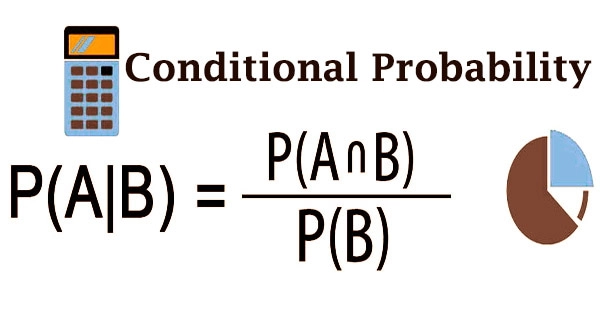Probability is essentially a measure of the possibility of something happening. And the likelihood ranges from 0 (impossible) to 1 (possible) (certain). In a sample space, the total of all probability for all events is 1. The likelihood of an event occurring if another event has already occurred .....

### Unconditional Probability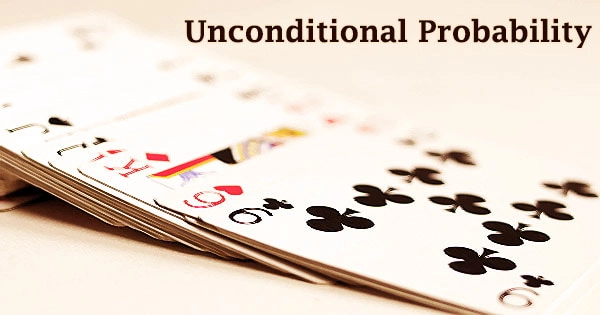Unconditional probability, often known as marginal probability, is the likelihood that one of the multiple alternative outcomes will occur. It’s a likelihood that isn’t influenced by past or future occurrences. Unlimited probability is the likelihood that an occasion will end with a p.....

### Joint Probability (JP)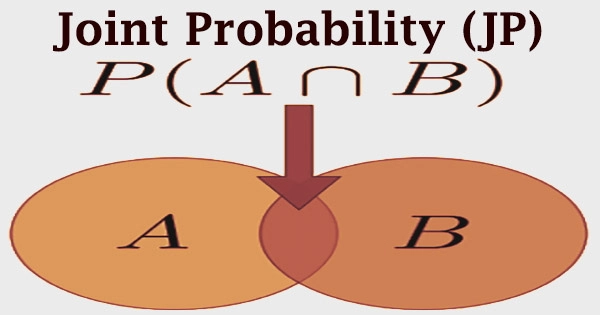In probability theory, a joint probability is a chance that two occurrences will happen at the same time. It’s a statistical metric that assesses the chances of two occurrences occurring at the same time and in the same place. To put it another way, the joint probability is the probability .....

### Subjective ProbabilitySubjective probabilities, as the name implies, are probabilities based on an individual’s personal assessment of the likelihood of an event occurring. It is not based on market data or historical data, and it is unique to each individual. As such, it is made from the assessment of an indivi.....

### Coefficient of Determination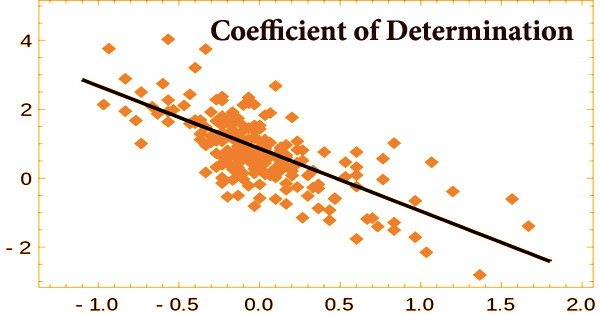In a regression model, the coefficient of determination (R2 or r-squared) is a statistical metric that determines how much variance in the dependent variable can be explained by the independent variable. It’s a statistic used in statistical models whose main goal is either to forecast futur.....

### Autocorrelation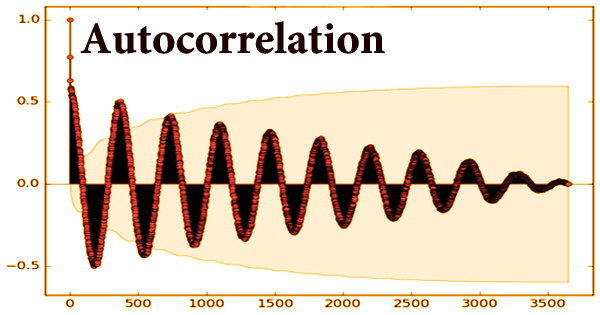In the discrete-time situation, autocorrelation, also known as serial correlation, refers to the degree of correlation between the values of the same variables across multiple observations in the data. It assesses the relationship between the lagged version of a variable’s value and the ori.....

### Correlation Coefficient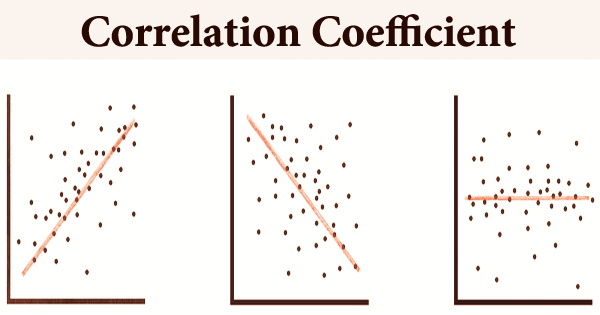A correlation coefficient is a statistical measure of how strong a link exists between two variables’ relative movements. Two columns of a given data set of observations referred to as a sample or two components of a multivariate random variable with a known distribution can be used as vari.....